HOME plateWON | World!OfNumbers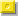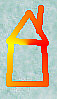Palindromic Numbersin other bases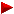Sum of First NumbertypesSequence ProductsReversal ProductsPythagorean TriplesPalindromes in ConcatenationsVarious Palindromic Sums

Introduction

Palindromic numbers are numbers which read the same fromleft to right (forwards) as from the right to left (backwards)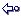Here are a few random examples : 7, 3113, 44611644

1. Go directly to the Base 2 topic
2. Go directly to the Base 3 topic
3. Go directly to the Base 12 topic
4. Go directly to the Base 16 topicBase 2Palindromes in bases 2 and 10.
The main source for this table comes from the following weblink.
Binary/Decimal Palindromes by Charlton Harrison (email) from Austin, Texas.

" I just finished writing a distributed client/server program for finding these numbers, and I currently
have it running on 4 different machines at the same time and I'm finding them A LOT faster. That's how
I was able to come up with those new ones. I'd say there are more to come in the near future, too."

 [ December 1, 2001 ]Charlton Harrison once found this record binary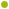decimal palindrome110 0010111100 0010101010 1101000011 10100000100000101110 0001011010 1010100001 11101000117475703079870789703075747The binary string contains 83 digits !

[ April 11, 2003 ]
Dw (email) wrote me the following :

"Using a backtracking solver, I have found larger numbers.
The first of these, which is the next after the one mentioned above, is
50824513851188115831542805 (86 bit, 3*5*11*11*17*17*83*11974799*97488286319).
There are no other 86 bit double palindromes.

I also have found two 89 bit double palindromes, but I am not sure if they
are the next ones. There may be some lower ones in 89 bit (haven't searched
that space completely yet) or in 87 bit that I haven't found.
These numbers are
532079161251434152161970235 (5*29*85839676103*42748430836381)
and
552963956270141072659369255 (5*7*7*71*441607*71984077228507867)
As can be seen by their factor representation, they are all composite."50824513851188115831542805 {26}
10101000001010100000100110101010101001100000000110010101010101100100000101010000010101 {86}532079161251434152161970235 {27}
11011100000100000001001010010000011001110101010101110011000001001010010000000100000111011 {89}552963956270141072659369255 {27}
11100100101100110101011000010001001111100100100100111110010001000011010101100110100100111 {89}

[ April 13, 2003 ]
Dw (email) found four new ones including the 6th double palindromic prime :

"They are, in opposite sorted order:
138758321383797383123857831 (87 bit, composite, the only 87 bit double palindrome)
390714505091666190505417093 (89 bit, prime)
351095331428353824133590153 (89 bit, composite)
795280629691202196926082597 (90 bit, composite, not sure if this is the next one)."

When asking Dw for an explanation or description in a few words
what he meant by 'Using a backtracking solver' he replied :

"A backtracking solver is one that solves a problem made up of smaller
problems by trying every one except if it knows its earlier guesses make all
later ones depending on them impossible.

For instance, if you're in a maze and know that all corridors with green
walls eventually lead to a dead end, you can turn around as soon as you find
such a wall (backtrack) if you're trying to find the exit.

The smaller problem is avoiding a dead end, and the larger one is
finding the exit.

If you want the details:

My general strategy goes that to find a double palindromic number, the
solution to its palindromic decimal representation minus its binary
representation must be zero (since they are equal).

Furthermore, you can write a decimal palindrome like 101 * a + 10 * b, where
a and b are digits. The same can be done for binary, and you end up with a
giant (linear diophantine) equation of positive decimal and negative binary factors.

The factors can then be solved, one at a time, using the extended euclidean
algorithm. These are the smaller problems.

I also have a table of maximum and minimum values for each step. That way, if
it's impossible for the binary factors left to subtract enough from the
decimal factors to get zero (or the other way around), the solver backtracks."

 [ April 13, 2003 ]Dw once found this record binarydecimal palindrome1010010001 1101011100 1110010101 0010100001 0100000010 1000010100 1010100111 0011101011 1000100101795280629691202196926082597The binary string contains 90 digits !The decimal string contains 27 digits !138758321383797383123857831 {27}
111001011000111001101111010110010111011100111001110111010011010111101100111000110100111 {87}351095331428353824133590153 {27}
10010001001101011010101000000110111100000100000100000111101100000010101011010110010001001 {89}390714505091666190505417093 {27}
10100001100110001000000111100001100011000111011100011000110000111100000010001100110000101 {89}795280629691202196926082597 {27}
101001000111010111001110010101001010000101000000101000010100101010011100111010111000100101 {90}

[ May 21, 2003 ]
Dw (email) found a new Binary/Decimal Palindrome :

"I have been trying to find a polynomial time (growth of time needed is a
polynomial of number of bits) algorithm for finding double palindromes of
base 2 and 10. I haven't succeeded yet (my backtracking solver being
exponential), but I have found some interesting things.

For one, to find double palindromes in base 2 and 8 is very simple. Each base 8
digit maps to three bits. Therefore, every double palindrome must consist
of digits who themselves are double palindromes.

For instance, 757 as well as 575 is double palindromic. (These values are 495
and 381 in decimal respectively).
5 maps to 101 in binary, and 7 to 111 in binary.

I have found 1609061098335005338901609061 (91 bits composite),
and this is the only 91 bit one. No 92 bit double palindrome exists, and
93 bits seems to require several days of searching; therefore I'm trying to
find a polynomial time algorithm as mentioned above.

Another approach could be to create a networked version (to do the search on
multiple computers), but I haven't done that yet."

 [ May 21, 2003 ]Dw once found this record binarydecimal palindrome1 0100110010 1111101111 1100001110 11001000000010001000 0000100110 1110000111 1110111110 1001100101 1609061098335005338901609061The binary palindrome contains 91 digits !The decimal string contains 28 digits !1609061098335005338901609061 {28}
1010011001011111011111100001110110010000000100010000000100110111000011111101111101001100101 {91}

[ June 12, 2003 ]
Dw (email) found new Binary/Decimal Palindromes :

"I rewrote my program to use another strategy at finding the numbers, and this
let me search somewhat faster. As a result, I have found binary/decimal
palindromes up to 102 bits -- broke the 100 bit barrier so to speak.

These are:

None at 92 or 93 bits.
17869806142184248124160896871 (94 bits)
19756291244127372144219265791 (94 bits)
30000258151173237115185200003 (95 bits)
30658464822225352222846485603 (95 bits)
56532345659072227095654323565 (96 bits)
None at 97 or 98 bits.
378059787464677776464787950873 (99 bits)
1115792035060833380605302975111 (100 bits)
None at 101 bits.
3390741646331381831336461470933 (102 bits)

There may be higher ones at 102 bits; I haven't completed the search there."17869806142184248124160896871 {29}
1110011011110110001110101001000001000000000011001100000000001000001001010111000110111101100111 {94}19756291244127372144219265791 {29}
1111111101011000000101011001100101101101100001111000011011011010011001101010000001101011111111 {94}30000258151173237115185200003 {29}
11000001110111110100001110001010010000110100011011000101100001001010001110000101111101110000011 {95}30658464822225352222846485603 {29}
11000110001000000010110011101000100010000101111111110100001000100010111001101000000010001100011 {95}56532345659072227095654323565 {29}
101101101010101001110101110101101111011010100000000001010110111101101011101011100101010101101101 {96}378059787464677776464787950873 {30}
100110001011001001110111010000000001110111000111010111000111011100000000010111011100100110100011001 {99}1115792035060833380605302975111 {31}
1110000101010101000110001001111111101011111110110110110111111101011111111001000110001010101010000111 {100}3390741646331381831336461470933 {31}
101010110011000001001111000000100001000111101001011110100101111000100001000000111100100000110011010101 {102}

[ June 17, 2003 ]

" There are no numbers for 103 bits."
[ Note : In fact there is one! See index number 97. PDG ]

 [ June 17, 2003 ]Dw once found this record binarydecimal palindrome10 1010110011 0000010011 1100000010 0001000111 10100101111010010111 1000100001 0000001111 0010000011 0011010101 3390741646331381831336461470933The binary palindrome contains 102 digits !The decimal string contains 31 digits !Binary/Decimal Palindromes by Charlton Harrison (email) : the longest list in existence ?

[ September 30, 2015 ]
See index numbers 124, 125 & 126.

 [ September 30, 2015 ]A former record binarydecimal palindrome 100110011011011101000001000010000011000111001011100111100011101000 00101110001111001110100111000110000010000100000101110110110011001 1634587141488515712882175158841417854361The binary palindrome contains 131 digits !The decimal string contains 40 digits !

[ March 8, 2020 ]
See index numbers up to 147. The record dates from the end of 2015.

 [ December 30, 2015 ]The current record binarydecimal palindrome Search team : Robert G. Wilson v, Charlton Harrison, Ilya Nikulshin & Andrey Astrelin 11010001010011101000000001001100010000000010110001011110100100101011100110101 0101100111010100100101111010001101000000001000110010000000010111001010001011 9335388324586156026843333486206516854238835339The binary palindrome contains 153 digits !The decimal string contains 46 digits !

IndexDecimal equivalent of
binary palindrome
A007632
Binary
length
1479335388324586156026843333486206516854238835339153
1465318935032766104711807997081174016672305398135152
145903240486809073407096959690704370908684042309150
144706400325289926993853434358399629982523004607149
143557019370941199612258585852216991149073910755149
1429101547767757547725021205277457577677451019143
1417342779513978827245484845427288793159772437143
1403381578420704603001613161003064070248751833142
1391816060344791285708869688075821974430606181141
138905357630732463833436634338364237036753509140
137595943598626320807905509708023626895349595139
136309612431907274418544445814472709134216903138
135170341815153453197154451791354351518143071137
134139035351443367699760067996763344153530931137
133128795669673344381770077183443376966597821137
13298801466348600079992129997000684366410889137
13156545858306667087923432978076660385854565136
13038090421176450233778487733205467112409083135
12931636759764024794204540249742046795763613135
12814327425216354951264146215945361252472341134
1277114907950920173924554293710290597094117133
1261634587141488515712882175158841417854361131
1251017421766189445102992015449816671247101130
124773609618198307097595790703891816906377130
123551700061998405245575542504899160007155129
122131674457014330218696812033410754476131127
121124192421350471300727003174053124291421127
120122240824002234545959545432200428042221127
11932190158233101105022050110133285109123125
1189970387454991896491946981994547830799123
1179707999142717984907094897172419997079123
1165893890080115984244424895110800983985123
1151681824725831390428240931385274281861121
1141323475457008895965695988007545743231120
113998021119318189842248981813911120899120
112794397832642722540045227246238793497120
111710084230446469950059964644032480017120
110139124355701640720027046107553421931117
10996754720977532710701723577902745769117
10894285848717805140304150871784858249117
10776759778311938325452383911387795767116
10654074940541725088788052714504947045116
10510827628430039640604693003482672801114
10410652099006552766666725560099025601114
1039932525402284695775964822045252399113
1021480869563960100770010693659680841111
1011409460884147943003497414880649041111
100579782100975917393719579001287975109
99332997156422555464555224651799233109
98188726493036450333054630394627881108
977155681676104835384016761865517103
963390741646331381831336461470933102
951115792035060833380605302975111100
9437805978746467777646478795087399
935653234565907222709565432356596
923065846482222535222284648560395
913000025815117323711518520000395
901975629124412737214421926579194
891786980614218424812416089687194
88160906109833500533890160906191
8779528062969120219692608259790
8655296395627014107265936925589
8553207916125143415216197023589
84Prime Curios!    39071450509166619050541709389
8335109533142835382413359015389
8213875832138379738312385783187
815082451385118811583154280586
80747570307987078970307574783
79726098868852025886889062783
78581298856301310365889218583
77121922815870107851822912181
76119431376139393167313491180
75113048607481718470684031180
749426180558383855081624977
739291340177595771043192977
727292808819585918808292776
711746199894868498991647174
7053947532817182357493569
6911435412612162145341167
689477815742247518774967
673288994178871499882365
661087974024420479780164
65967486872327868476964
64703626712621762630763
6331355815335185531359
6216120615225160216158
615595263707362595556
603762992707299267356
593707879686978707356
583410448202844014355
571827944080449728155
561081967191769180154
551045758747857540154
54314895577559841352
53179377077077397151
5293313836383133950
5155221253521225549
503414138831414345
49948487478484944
48Prime Curios!    728471717482743
47722752625722743
46565262226256543
45199992529999141
44179409690497141
43179270407297141
42147492229474141
41141389998314141
40123410401432141
3913652552563137
3811094884901137
377501515105737
363247929742335
351846212648135
341005090500134
33745111154733
32129088092131
3193947493930
3091037301930
2971984891730
281350053124
27584148523
26525952523
25507170523
24312921322
23197979121
22193439121
21175857121
2058558520
197373717
185383516
175323516
163999316
153222315
141535114
13900914
12744713
1171710
1058510
9Prime Curios!    3139
8997
7336
694
5Prime!    73
4Prime!    53
3Prime!    32
211
101

Now that we entered the world of binary numbers let's find out more about Palindromic Squares in base 2.
I retrieved these from Sloane's table namely entry number A003166 !

IndexBasenumber
(base 10)
Square
(base 10)
Palindromic Square
(base 2)
331178448744881657138874144431315270535493506564910001100001110100001111111111101000110011111110110101101111111001100010111111111110000101110000110001
( Discovered by Don Knuth ) [ 101 ]
32301429589329949908597973236217040307883426011001001011001010101110110011110100111111000111111111110001111110010111100110111010101001101001001
( Discovered by Don Knuth ) [ 97 ]
31285000288617375812251645119870499891518906251000001100111001111100001100111110011100000101101011010000011100111110011000011111001110011000001
( Discovered by Don Knuth ) [ 97 ]
301547374339040912394367345122293089418653628110011010101110110111110000100101010011110101100000110101111001010100100001111101101110101011001
( Discovered by Jon E. Schoenfield ) [ 95 ]
293919294370597315360868363395685954758767291001111011010011111000010100100000100011000111110001100010000010010100001111100101101111001
( Discovered by Jon E. Schoenfield ) [ 91 ]
2825100748703521630047585477311162357797441100000100100101001110001000000110000010100011110001010000011000000100011100101001001000001
( Discovered by Jon E. Schoenfield ) [ 90 ]
271860026448759134596983900833888579298328110001111000101101111101101000110110101001010101010010101101100010110111110110100011110001
( Discovered by Jon E. Schoenfield ) [ 89 ]
261824203895099933277198508976469632309800110001001101000011001101010111010101110110000000001101110101011101010110011000010110010001
( Discovered by Jon E. Schoenfield ) [ 89 ]
25128633643602971654661426657590480299282091000100011011110110100011001000101001010111111110101001010001001100010110111101100010001
( Discovered by Jon E. Schoenfield ) [ 88 ]
24927427835701786012239043433944913138289100011100100101110011001101001001010001110111011100010100100101100110011101001001110001
( Discovered by Jon E. Schoenfield ) [ 87 ]
234902182461643240313928872402231662594491001111100000110110000110101100110111001000001001110110011010110000110110000011111001
( Discovered by Jon E. Schoenfield ) [ 85 ]
22161545264280726096872411521207288392491000101000100111110110110111000111000010000100001110001110110110111110010001010001
( Discovered by Jon E. Schoenfield ) [ 82 ]
21202491428745410027787151914122750251000101011101100001111011100110011110110111100110011101111000011011101010001
( Discovered by Jon E. Schoenfield ) [ 76 ]
201071374004751147842258054053022562510011011100011111100011100111010011011011001011100111000111111000111011001
( Discovered by Jon E. Schoenfield ) [ 74 ]
191016573439931033421558771113318404910001100000011100000001101000101000000000010100010110000000111000000110001
( Discovered by Jon E. Schoenfield ) [ 74 ]
187379805072354461522905144808227291001001110011110010001011101111010100010101111011101000100111100111001001
( Discovered by Jon E. Schoenfield ) [ 73 ]
1717360493407301386731334490467649100000101011010010100110000110101010101011000011001010010110101000001
( Discovered by Jon E. Schoenfield ) [ 69 ]
1647705049392275771737302339372110011101111010011101001110110000000001101110010111001011110111001
( Discovered by Patrick De Geest ) [ 65 ]
1554295919929480469177872160110000010111010110110100111000100011100101101101011101000001
[ 59 ]
143901178731521919548340441291000011100101100011100011011001101100011100011010011100001
[ 58 ]
1329574243787463589042698969100110110101110111010101110111011101010111011101011011001
[ 57 ]
1268301841466514148398928110000100100101110101111100100111110101110100100100001
[ 53 ]
11179064993206427064370011001000111001111101110010100111011111001110001001
[ 49 ]
1012489657155991531977649100011011101111110011011110110011111101110110001
[ 48 ]
96435309414132019254811001011010101001000011001100001001010101101001
[ 46 ]
831680991003685127380110010010000011100010111101000111000001001001
[ 44 ]
71092489119353221512110001010111100100000100000100111101010001
[ 41 ]
61416832007407248910010101100100000100000100110101001
[ 35 ]
51819733113080910011101111001010011110111001
[ 29 ]
4119911437840811000100100011111100010010001
[ 28 ]
34523204575291001110000010100000111001
[ 25 ]
239101
[ 3 ]
1111
[ 1 ]Base 3 and base 9You all probably noticed that I 'restrict' myself searching for large palindromes mainly in the decimal numbersystem.
Not so for Kevin Brown ! Here are a few palindromic tetrahedrals that he discovered in other base representations.

formula = k(k+1)(k+2)/6
kin base 3in base 9
111
2114
310111
420222
62002
12111111444
13 555
14202202
39112121211
120 488884
392201000222000102
4961102111111112011
588 71066017
1093 505555505
88573 505055555550505Base 12Alain Bex (email) gave also some palindromic squares in base 12 :

Note that again some of the basenumbers are palindromic themselves
and share many similarities with the palindromic squares in base 10 !
See sequence with index number A029737 in Sloane's table for these numbers.

IndexDecimal
equivalent
Basenumber
(base 12)
Palindromic Square
(base 12)
5754480422334449635653694
5651841421001244104A40144
5550486820420441496869414
5449953820110240441A14404
5349766620000240000800004
5230812512A39116497679461
5129032912002114404A40441
5028876711B13B141B1B1B141
4927145311111112345654321
4826958111001112102420121
4725257710220110444A44401
4625070510110110221412201
4524883310000110000200001
4488829434A51642662461
436399931053963848369
424541222344496787694
4143358211124456B6544
4043214210124414A4144
394190820304410212014
384161820102404090404
374147420002400080004
3628613146851AA222AA1
352557912977163151361
34255231292B1621B1261
33245991229B14A797A41
3224361121211468B8641
3124217120211444A4441
302407311B111420B0241
292276511211125686521
282262111111123454321
272247711011121242121
2621169103011060B0601
252102510201104060401
242088110101102030201
232073710001100020001
223796224449AAA94
2136142112445A544
20345820024008004
1920411221148A841
18188511111234321
17178310471093901
1617451015102A201
15172910011002001
1461142B160061
1330221244944
1229220441414
1129020240804
1018113116B61
917912B16661
816912114641
715711112321
614510110201
52622484
41311121
3339
2224
1111Base 16[ December 28, 2008 ]
Matt S.
asked himself how difficult is it to generate the elements of these sequences ?

as he has derived e.g. 1152921504606846977 that's well beyond what
you have listed.

Here is my reply after some perusing (PDG):

I found

10485773 = 1152924803144876033 = 1000030000300001{16}

11529215046068469773 = 1532495540865888862346031014505056805788924816845176833 =
1000000000000003000000000000003000000000000001{16}

Is this how you derived the number by working backwards from
the pattern 1_0[x]_3_0[x]_3_0[x]_1 you discovered ?
Anyway well done and congratulations!

Ten years ago I submitted my sequences. So forgive me that I didn't
kept my original code. But from recollection I just searched in
a straightforward manner with the UBASIC program from zero till
I got tired with the last entry. Modern fast computers on the other
hand should have no problem in recreating the known sequences numbers
from A029735 & A029736.
Of course your number cannot yet be added as there might be other
solutions in between very probably.

Matt S. wrote :

The largest number I have right now is approx 281 bits. I have *many*
more too. Apologies for the rudimentary notation - this is all fairly
new to me. I'm actually generating these numbers with a friend, all
coming from a coded letter that was sent to fermilab
http://www.symmetrymagazine.org/breaking/2008/05/15/code-crackers-wanted/
I have little to no training in this area.

1_0[x]_3_0[x]_3_0[x]_1 is correct for the power of 3

It can be extended into other powers as well. For example :
['1', '0', '4', '0', '6', '0', '4', '0', '1']
and on and on.

I'd like to track down where the aforementioned letter came from - and
how this sequence is involved. Surely not a coincidence ? Any ideas ?
I'm eager to hear what your thoughts are regarding the letter / sequence.
As I said, I realize it's an odd request... so any insight is welcome.

Sources RevealedBinary/Decimal Palindromes by Charlton Harrison (email) : the longest list in existence ?

 Neil Sloane's "Integer Sequences" Encyclopedia can be consulted online : Neil Sloane's Integer Sequences I sampled the following base X palindromic numbers sequences from the table : %N Binary expansion is palindromic. under A006995 -- Sum of digits A043261 %N Palindromes in base 3 (written in base 10). under A014190 -- Sum of digits A043262 %N Palindromes in base 4 (written in base 10). under A014192 -- Sum of digits A043263 %N Palindromic in base 5. under A029952 -- Sum of digits A043264 %N Palindromic in base 6. under A029953 -- Sum of digits A043265 %N Palindromic in base 7. under A029954 -- Sum of digits A043266 %N Palindromic in base 8. under A029803 -- Sum of digits A043267 %N Palindromic in base 9. under A029955 -- Sum of digits A043268 %N Palindromes. under A002113 -- Sum of digits A043269 %N Palindromic in base 11. under A029956 -- Sum of digits A043270 %N Palindromic in base 12. under A029957 -- Sum of digits A043271 %N Palindromic in base 13. under A029958 -- Sum of digits A043272 %N Palindromic in base 14. under A029959 -- Sum of digits A043273 %N Palindromic in base 15. under A029960 -- Sum of digits A043274 %N Palindromic in base 16. under A029730 -- Sum of digits A043275 %N Palindromic in bases 2 and 3. under A060792. %N Palindromic in bases 2 and 10. under A007632. %N Palindromic in bases 3 and 10. under A007633. %N Palindromic in bases 4 and 10. under A029961. %N Palindromic in bases 5 and 10. under A029962. %N Palindromic in bases 6 and 10. under A029963. %N Palindromic in bases 7 and 10. under A029964. %N Palindromic in base 8 and base 10. under A029804. %N Palindromic in bases 9 and 10. under A029965. %N Palindromic in bases 11 and 10. under A029966. %N Palindromic in bases 12 and 10. under A029967. %N Palindromic in bases 13 and 10. under A029968. %N Palindromic in bases 14 and 10. under A029969. %N Palindromic in bases 15 and 10. under A029970. %N Square in base 2 is a palindrome. under A003166. %N Squares which are palindromes in base 2. under A029983. %N n^2 is palindromic in base 3. under A029984. %N Squares which are palindromic in base 3. under A029985. %N n^2 is palindromic in base 4. under A029986. %N Squares which are palindromic in base 4. under A029987. %N n^2 is palindromic in base 5. under A029988. %N Squares which are palindromic in base 5. under A029989. %N n^2 is palindromic in base 6. under A029990. %N Squares which are palindromic in base 6. under A029991. %N n^2 is palindromic in base 7. under A029992. %N Squares which are palindromic in base 7. under A029993. %N n^2 is palindromic in base 8. under A029805. %N n in base 8 is a palindromic square. under A029806. %N n^2 is palindromic in base 9. under A029994. %N Squares which are palindromic in base 9. under A029995. %N Square is a palindrome. under A002778. %N Palindromic Squares. under A002779. %N n^2 is palindromic in base 11. under A029996. %N Squares which are palindromic in base 11. under A029997. %N n^2 is palindromic in base 12. under A029737. %N Squares which are palindromic in base 12. under A029738. %N n^2 is palindromic in base 13. under A029998. %N Squares which are palindromic in base 13. under A029999. %N n^2 is palindromic in base 14. under A030072. %N Squares which are palindromes in base 14. under A030074. %N n^2 is palindromic in base 15. under A030073. %N Squares which are palindromes in base 15. under A030075. %N n^2 is palindromic in base 16. under A029733. %N Palindromic squares in base 16. under A029734. %N n^3 is palindromic in base 4. under A046231. %N Cubes which are palindromes in base 4. under A046232. %N n^3 is palindromic in base 5. under A046233. %N Cubes which are palindromes in base 5. under A046234. %N n^3 is palindromic in base 6. under A046235. %N Cubes which are palindromes in base 6. under A046236. %N n^3 is palindromic in base 7. under A046237. %N Cubes which are palindromes in base 7. under A046238. %N n^3 is palindromic in base 8. under A046239. %N Cubes which are palindromes in base 8. under A046240. %N n^3 is palindromic in base 9. under A046241. %N Cubes which are palindromes in base 9. under A046242. %N Cube is a palindrome. under A002780. %N Palindromic cubes. under A002781. %N n^3 is palindromic in base 11. under A046243. %N Cubes which are palindromes in base 11. under A046244. %N n^3 is palindromic in base 12. under A046245. %N Cubes which are palindromes in base 12. under A046246. %N n^3 is palindromic in base 13. under A046247. %N Cubes which are palindromes in base 13. under A046248. %N n^3 is palindromic in base 14. under A046249. %N Cubes which are palindromes in base 14. under A046250. %N n^3 is palindromic in base 15. under A046251. %N Cubes which are palindromes in base 15. under A046252. %N n^3 is palindromic in base 16. under A029735. %N Cubes which are palindromes in base 16. under A029736. %N Palindromic primes in base 2. under A016041. %N Palindromic primes in base 3. under A029971. %N Palindromic primes in base 4. under A029972. %N Palindromic primes in base 5. under A029973. %N Palindromic primes in base 6. under A029974. %N Palindromic primes in base 7. under A029975. %N Palindromic primes in base 8. under A029976. %N Octal palindromes which are also primes. under A006341. %N Palindromic primes in base 9. under A029977. %N Palindromic primes. under A002385. %N Palindromic primes in base 11. under A029978. %N Palindromic primes in base 12. under A029979. %N Palindromic primes in base 13. under A029980. %N Palindromic primes in base 14. under A029981. %N Palindromic primes in base 15. under A029982. %N Palindromic primes in base 16. under A029732. %N Palindromic primes in base 10 and base 2. under A046472. %N Palindromic primes in base 10 and base 3. under A046473. %N Palindromic primes in base 10 and base 4. under A046474. %N Palindromic primes in base 10 and base 6. under A046475. %N Palindromic primes in base 10 and base 7. under A046476. %N Palindromic primes in base 10 and base 8. under A046477. %N Palindromic primes in base 10 and base 9. under A046478. %N Palindromic primes. under A002385. %N Palindromic primes in base 10 and base 11. under A046479. %N Palindromic primes in base 10 and base 12. under A046480. %N Palindromic primes in base 10 and base 13. under A046481. %N Palindromic primes in base 10 and base 14. under A046482. %N Palindromic primes in base 10 and base 15. under A046483. %N Palindromic primes in base 10 and base 16. under A046484. %N Palindromic primes in bases 2 and 4. under A056130. %N Palindromic primes in bases 2 and 8. under A056145. %N Palindromic primes in bases 4 and 8. under A056146. %N Not palindromic in any base from 2 to n-2. under A016038. %N Smallest palindrome greater than n in bases n and n+1. under A048268. %N First palindrome greater than n+2 in bases n+2 and n. under A048269. %N The first non-trivial (k>n+2) palindromic prime in both bases n and n+2. under A057199. %N Symmetric bit strings (bit-reverse palindromes), including as many leading as trailing zeros. under A057890. Click here to view some of the author's [P. De Geest] entries to the table. Click here to view some entries to the table about palindromes.

More Integer Sequences from Sloane's OEIS database

 A043001 n-th base 3 palindrome that starts with 1. - Clark Kimberling A043002 n-th base 3 palindrome that starts with 2. - Clark Kimberling A043003 n-th base 4 palindrome that starts with 1. - Clark Kimberling A043004 n-th base 4 palindrome that starts with 2. - Clark Kimberling A043005 n-th base 4 palindrome that starts with 3. - Clark Kimberling A043006 n-th base 5 palindrome that starts with 1. - Clark Kimberling A043007 n-th base 5 palindrome that starts with 2. - Clark Kimberling A043008 n-th base 5 palindrome that starts with 3. - Clark Kimberling A043009 n-th base 5 palindrome that starts with 4. - Clark Kimberling A043010 n-th base 6 palindrome that starts with 1. - Clark Kimberling A043011 n-th base 6 palindrome that starts with 2. - Clark Kimberling A043012 n-th base 6 palindrome that starts with 3. - Clark Kimberling A043013 n-th base 6 palindrome that starts with 4. - Clark Kimberling A043014 n-th base 6 palindrome that starts with 5. - Clark Kimberling A043015 n-th base 7 palindrome that starts with 1. - Clark Kimberling A043016 n-th base 7 palindrome that starts with 2. - Clark Kimberling A043017 n-th base 7 palindrome that starts with 3. - Clark Kimberling A043018 n-th base 7 palindrome that starts with 4. - Clark Kimberling A043019 n-th base 7 palindrome that starts with 5. - Clark Kimberling A043020 n-th base 7 palindrome that starts with 6. - Clark Kimberling A043021 n-th base 8 palindrome that starts with 1. - Clark Kimberling A043022 n-th base 8 palindrome that starts with 2. - Clark Kimberling A043023 n-th base 8 palindrome that starts with 3. - Clark Kimberling A043024 n-th base 8 palindrome that starts with 4. - Clark Kimberling A043025 n-th base 8 palindrome that starts with 5. - Clark Kimberling A043026 n-th base 8 palindrome that starts with 6. - Clark Kimberling A043027 n-th base 8 palindrome that starts with 7. - Clark Kimberling A043028 n-th base 9 palindrome that starts with 1. - Clark Kimberling A043029 n-th base 9 palindrome that starts with 2. - Clark Kimberling A043030 n-th base 9 palindrome that starts with 3. - Clark Kimberling A043031 n-th base 9 palindrome that starts with 4. - Clark Kimberling A043032 n-th base 9 palindrome that starts with 5. - Clark Kimberling A043033 n-th base 9 palindrome that starts with 6. - Clark Kimberling A043034 n-th base 9 palindrome that starts with 7. - Clark Kimberling A043035 n-th base 9 palindrome that starts with 8. - Clark Kimberling A043036 n-th base 10 palindrome that starts with 1. - Clark Kimberling A043037 n-th base 10 palindrome that starts with 2. - Clark Kimberling A043038 n-th base 10 palindrome that starts with 3. - Clark Kimberling A043039 n-th base 10 palindrome that starts with 4. - Clark Kimberling A043040 n-th base 10 palindrome that starts with 5. - Clark Kimberling A043041 n-th base 10 palindrome that starts with 6. - Clark Kimberling A043042 n-th base 10 palindrome that starts with 7. - Clark Kimberling A043043 n-th base 10 palindrome that starts with 8. - Clark Kimberling A043044 n-th base 10 palindrome that starts with 9. - Clark Kimberling A043045 a(n)=(s(n)+2)/3, where s(n)=n-th base 3 palindrome that starts with 1. - Clark Kimberling A043046 a(n)=(s(n)+1)/3, where s(n)=n-th base 3 palindrome that starts with 2. - Clark Kimberling A043047 a(n)=(s(n)+3)/4, where s(n)=n-th base 4 palindrome that starts with 1. - Clark Kimberling A043048 a(n)=(s(n)+2)/4, where s(n)=n-th base 4 palindrome that starts with 2. - Clark Kimberling A043049 a(n)=(s(n)+1)/4, where s(n)=n-th base 4 palindrome that starts with 3. - Clark Kimberling A043050 a(n)=(s(n)+4)/5, where s(n)=n-th base 5 palindrome that starts with 1. - Clark Kimberling A043051 a(n)=(s(n)+3)/5, where s(n)=n-th base 5 palindrome that starts with 2. - Clark Kimberling A043052 a(n)=(s(n)+2)/5, where s(n)=n-th base 5 palindrome that starts with 3. - Clark Kimberling A043053 a(n)=(s(n)+1)/5, where s(n)=n-th base 5 palindrome that starts with 4. - Clark Kimberling A043054 a(n)=(s(n)+5)/6, where s(n)=n-th base 6 palindrome that starts with 1. - Clark Kimberling A043055 a(n)=(s(n)+4)/6, where s(n)=n-th base 6 palindrome that starts with 2. - Clark Kimberling A043056 a(n)=(s(n)+3)/6, where s(n)=n-th base 6 palindrome that starts with 3. - Clark Kimberling A043057 a(n)=(s(n)+2)/6, where s(n)=n-th base 6 palindrome that starts with 4. - Clark Kimberling A043058 a(n)=(s(n)+1)/6, where s(n)=n-th base 6 palindrome that starts with 5. - Clark Kimberling A043059 a(n)=(s(n)+6)/7, where s(n)=n-th base 7 palindrome that starts with 1. - Clark Kimberling A043060 a(n)=(s(n)+5)/7, where s(n)=n-th base 7 palindrome that starts with 2. - Clark Kimberling A043061 a(n)=(s(n)+4)/7, where s(n)=n-th base 7 palindrome that starts with 3. - Clark Kimberling A043062 a(n)=(s(n)+3)/7, where s(n)=n-th base 7 palindrome that starts with 4. - Clark Kimberling A043063 a(n)=(s(n)+2)/7, where s(n)=n-th base 7 palindrome that starts with 5. - Clark Kimberling A043064 a(n)=(s(n)+1)/7, where s(n)=n-th base 7 palindrome that starts with 6. - Clark Kimberling A043065 a(n)=(s(n)+7)/8, where s(n)=n-th base 8 palindrome that starts with 1. - Clark Kimberling A043066 a(n)=(s(n)+6)/8, where s(n)=n-th base 8 palindrome that starts with 2. - Clark Kimberling A043067 a(n)=(s(n)+5)/8, where s(n)=n-th base 8 palindrome that starts with 3. - Clark Kimberling A043068 a(n)=(s(n)+4)/8, where s(n)=n-th base 8 palindrome that starts with 4. - Clark Kimberling A043069 a(n)=(s(n)+3)/8, where s(n)=n-th base 8 palindrome that starts with 5. - Clark Kimberling A043070 a(n)=(s(n)+2)/8, where s(n)=n-th base 8 palindrome that starts with 6. - Clark Kimberling A043071 a(n)=(s(n)+1)/8, where s(n)=n-th base 8 palindrome that starts with 7. - Clark Kimberling A043072 a(n)=(s(n)+8)/9, where s(n)=n-th base 9 palindrome that starts with 1. - Clark Kimberling A043073 a(n)=(s(n)+7)/9, where s(n)=n-th base 9 palindrome that starts with 2. - Clark Kimberling A043074 a(n)=(s(n)+6)/9, where s(n)=n-th base 9 palindrome that starts with 3. - Clark Kimberling A043075 a(n)=(s(n)+5)/9, where s(n)=n-th base 9 palindrome that starts with 4. - Clark Kimberling A043076 a(n)=(s(n)+4)/9, where s(n)=n-th base 9 palindrome that starts with 5. - Clark Kimberling A043077 a(n)=(s(n)+3)/9, where s(n)=n-th base 9 palindrome that starts with 6. - Clark Kimberling A043078 a(n)=(s(n)+2)/9, where s(n)=n-th base 9 palindrome that starts with 7. - Clark Kimberling A043079 a(n)=(s(n)+1)/9, where s(n)=n-th base 9 palindrome that starts with 8. - Clark Kimberling A043080 a(n)=(s(n)+9)/10, where s(n)=n-th base 10 palindrome that starts with 1. - Clark Kimberling A043081 a(n)=(s(n)+8)/10, where s(n)=n-th base 10 palindrome that starts with 2. - Clark Kimberling A043082 a(n)=(s(n)+7)/10, where s(n)=n-th base 10 palindrome that starts with 3. - Clark Kimberling A043083 a(n)=(s(n)+6)/10, where s(n)=n-th base 10 palindrome that starts with 4. - Clark Kimberling A043084 a(n)=(s(n)+5)/10, where s(n)=n-th base 10 palindrome that starts with 5. - Clark Kimberling A043085 a(n)=(s(n)+4)/10, where s(n)=n-th base 10 palindrome that starts with 6. - Clark Kimberling A043086 a(n)=(s(n)+3)/10, where s(n)=n-th base 10 palindrome that starts with 7. - Clark Kimberling A043087 a(n)=(s(n)+2)/10, where s(n)=n-th base 10 palindrome that starts with 8. - Clark Kimberling A043088 a(n)=(s(n)+1)/10, where s(n)=n-th base 10 palindrome that starts with 9. - Clark Kimberling

1. A016038 Strictly non-palindromic numbers: n is not palindromic in any base b with 2 <= b <= n-2. - N. J. A. Sloane.
2. A100563 Number of bases less than sqrt(n) in which n is a palindrome. - Gordon Robert Hamilton

ContributionsOn General Palindromic NumbersAlain Bex (email) sent me the first palindromic squares in base 12 - go to topic.

Dw (email) found several binary/decimal palindromes of record lengths - go to topic.

[TOP OF PAGE]

Patrick De Geest - Belgium- Short Bio - Some Pictures